New AVERAGE Functions in Excel 2007

In this article, you’ll learn about the Average functions in Microsoft Excel. Average functions we use when we want to judge the overall group performance.  In Excel we can calculate average on the basis of numbers, criteria even more than one criteria. We have 4 types of Average functions in Microsoft Excel:-

• AVERAGE
• AVERAGEA
• AVERAGEIF
• AVERAGEIFS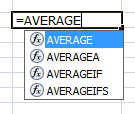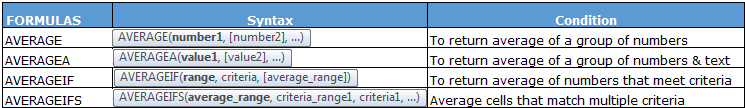AVERAGE Function:-

We use Average function to get the average of a group of numbers.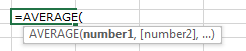Let’s take an example and understand:-

We have student’s score data, in which we want to calculate average score.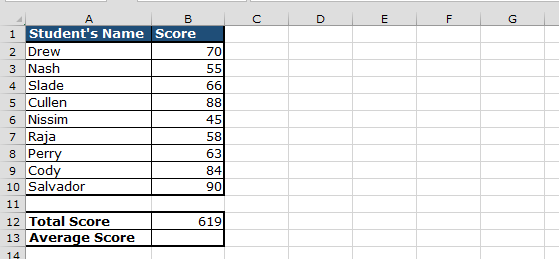• Enter the formula in cell B13
• =AVERAGE(B2:B10)
• Press Enter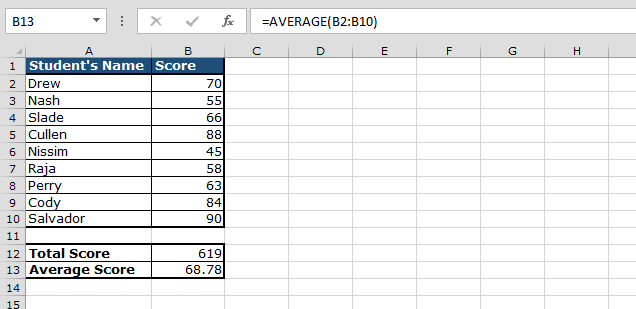AVERAGEA Function:-

We use this function to get the average of a group of numbers and Text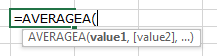Let’s take an example and understand:-

We have student’s scores, and also, 2 students were absent on the day of examination. Now, we want to calculate average score for all students including those who were absent.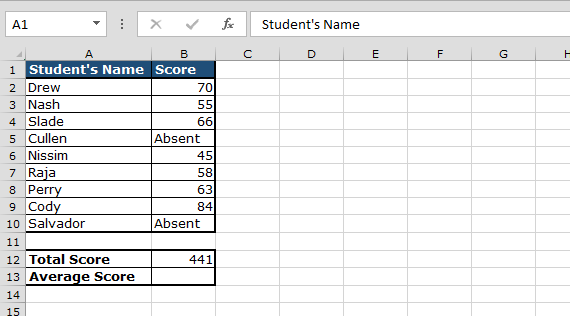• Enter the formula in cell B13
• =AVERAGEA(B2:B10)
• Press Enter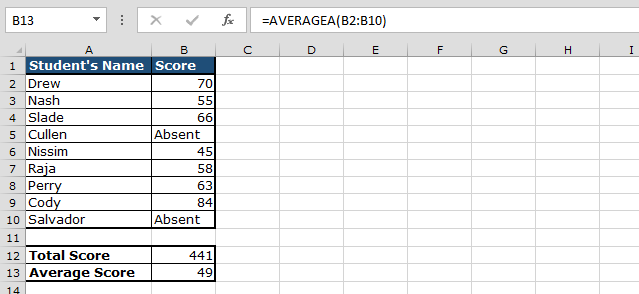AVERAGEIF Function

AVERAGEIF function is used to return the average on the basis of a criterion.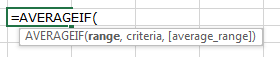Let’s take an example and understand:-

Similarly, we have student’s score data, and we want to calculate average numbers for those students who scored more than50 marks.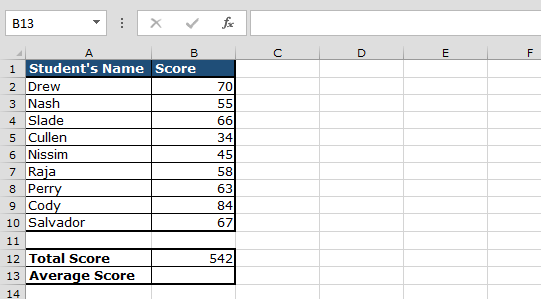• Enter the formula in cell B13
• =AVERAGEIF(B2:B10,”>50″,B2:B10)
• Press Enter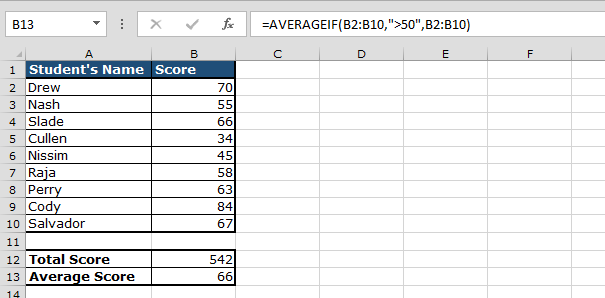AVERAGEIFS Function

AVERAGEIFS function is used to return the average on the basis of multiple criteria.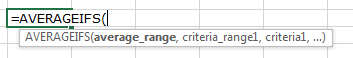Let’s take an example and understand

We have student’s scores semester-wise for every subject. Now, we want to calculate Average score in every semester for every student for every subject.

In data, we have drop down list of Student and, on the selection of Subject, average score should be changed accordingly.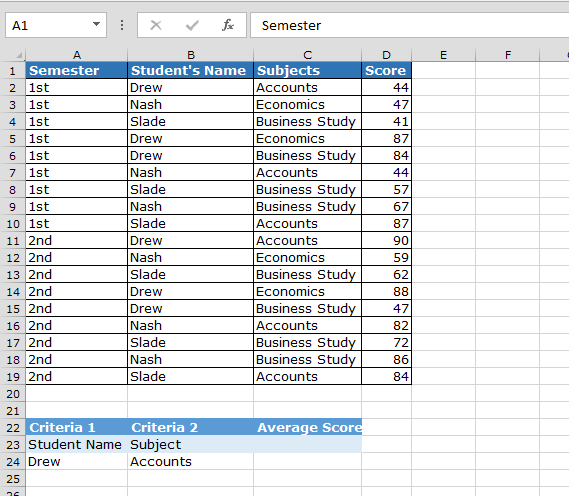• Enter the formula in cell B13
• =AVERAGEIFS(D2:D19,B2:B19,A24,C2:C19,B24)
• Press Enter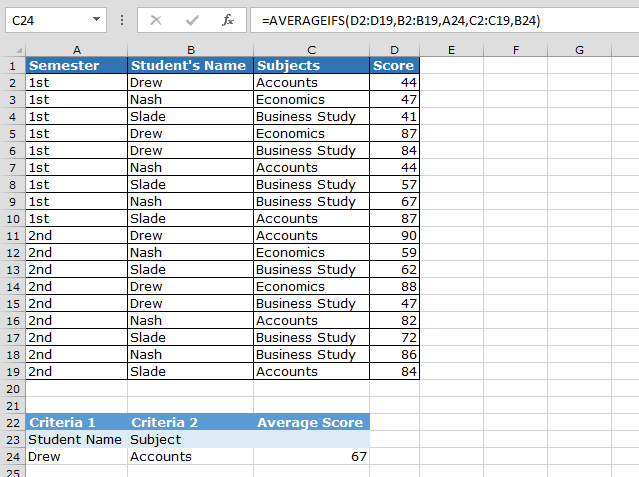Key take away:-

• Average family functions
• Uses of Average functions
• Comparison between AVERAGE and AVERAGEA function
• Comparison between AVERAGEIF and AVERAGEIFS function